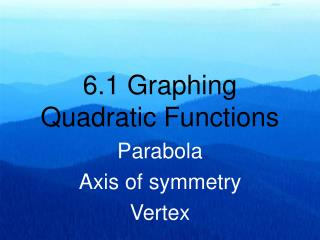DownloadDownload PresentationTélécharger la présentation- - - - - - - - - - - - - - - - - - - - - - - - - - - E N D - - - - - - - - - - - - - - - - - - - - - - - - - - -
##### Presentation Transcript

1. 6.1 Graphing Quadratic Functions Parabola Axis of symmetry Vertex

3. A Quadratic function The graph of a Quadratic function is called a parabola.

4. Parabola are Symmetrical Axis of Symmetry, splits it down the middle

5. Parabola are Symmetrical Points reflect across the axis of symmetry

6. Parabola are Symmetrical The equation for the axis symmetry is

7. The y – Intercept of a parabola If x = 0, then c is the y intercept

8. The Vertex of the Parabola The Vertex is a point at the highest or lowest point of the graph of a parabola. The Vertex is on the axis of symmetry, so its x coordinate is found by

9. How can you tell if the Vertex is the highest or lowest point. It all depends on “a”. If a > 0, the parabola If a<0, the parabola is opens upward. opens downward

10. The Maximum or Minimum value is the y value of the vertex If the vertex is ( -3, 1), of f(x)= x2 + 6x + 10, then the minimum value is 1. Since the parabola is opening upward it is the minimum.

11. How to graph the parabolaf(x) = 2- 4x + x2 Rewrite the function. f(x) = x2 -4x + 2 Find the y intercept: f(0) = 02 -4(0) + 2 = 2 (0, 2) Find the vertex: a = 1, b= -4

12. How to graph the parabolaf(x) = 2- 4x + x2 Start a table using number higher and lower then 2, from the vertex. Plot points

13. How to graph the parabolaf(x) = 2- 4x + x2 Connects the points.

14. Graph the function. Show the y intercept, axis of symmetry and vertex f(x) = -x2 + 2x + 3

15. Graph the function. Show the y intercept, axis of symmetry and vertex f(x) = -x2 + 2x + 3 Does the graph open up or down? What are a , b and c?

16. Graph the function. Show the y intercept, axis of symmetry and vertex f(x) = -x2 + 2x + 3 Does the graph open up or down? Down What are a , b and c? a = -1 b = 2 c = 3, so the y intercept is (0,3)

17. Graph the function. Show the y intercept, axis of symmetry and vertex f(x) = -x2 + 2x + 3 axis of symmetry is x = 1 What are a , b and c? a = -1 The vertex is b = 2 c = 3

18. Graph the function. Show the y intercept, axis of symmetry and vertex f(x) = -x2 + 2x + 3 Plot the points

19. Graph the function. Show the y intercept, axis of symmetry and vertex f(x) = -x2 + 2x + 3 Connect the points

20. Homework Page 291 # 15, 19, 23, 25, 33 – 43 odd

21. Homework Page 291- 292 # 16, 22, 26, 32 - 42 even, 46, 47